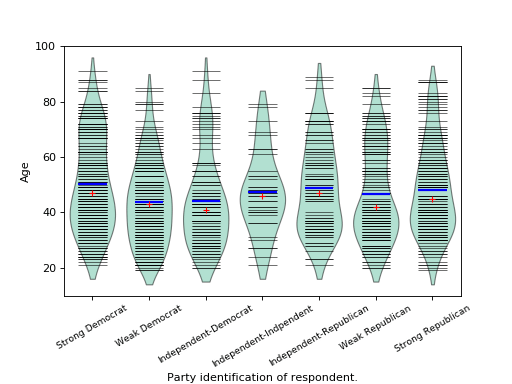/Statsmodels

# statsmodels.graphics.boxplots.beanplot

`statsmodels.graphics.boxplots.beanplot(data, ax=None, labels=None, positions=None, side='both', jitter=False, plot_opts={})` [source]

Make a bean plot of each dataset in the `data` sequence.

A bean plot is a combination of a `violinplot` (kernel density estimate of the probability density function per point) with a line-scatter plot of all individual data points.

Parameters: data (sequence of ndarrays) – Data arrays, one array per value in `positions`. ax (Matplotlib AxesSubplot instance, optional) – If given, this subplot is used to plot in instead of a new figure being created. labels (list of str, optional) – Tick labels for the horizontal axis. If not given, integers `1..len(data)` are used. positions (array_like, optional) – Position array, used as the horizontal axis of the plot. If not given, spacing of the violins will be equidistant. side ({'both', 'left', 'right'}, optional) – How to plot the violin. Default is ‘both’. The ‘left’, ‘right’ options can be used to create asymmetric violin plots. jitter (bool, optional) – If True, jitter markers within violin instead of plotting regular lines around the center. This can be useful if the data is very dense. plot_opts (dict, optional) – A dictionary with plotting options. All the options for `violinplot` can be specified, they will simply be passed to `violinplot`. Options specific to `beanplot` are: `’violin_width’ : float. Relative width of violins. Max available` space is 1, default is 0.8. ’bean_color’, MPL color. Color of bean plot lines. Default is ‘k’. Also used for jitter marker edge color if `jitter` is True. ’bean_size’, scalar. Line length as a fraction of maximum length. Default is 0.5. ’bean_lw’, scalar. Linewidth, default is 0.5. ’bean_show_mean’, bool. If True (default), show mean as a line. ’bean_show_median’, bool. If True (default), show median as a marker. ’bean_mean_color’, MPL color. Color of mean line. Default is ‘b’. ’bean_mean_lw’, scalar. Linewidth of mean line, default is 2. ’bean_mean_size’, scalar. Line length as a fraction of maximum length. Default is 0.5. ’bean_median_color’, MPL color. Color of median marker. Default is ‘r’. ’bean_median_marker’, MPL marker. Marker type, default is ‘+’. `’jitter_marker’, MPL marker. Marker type for jitter=True.` Default is ‘o’. ’jitter_marker_size’, int. Marker size. Default is 4. ’jitter_fc’, MPL color. Jitter marker face color. Default is None. ’bean_legend_text’, str. If given, add a legend with given text. fig – If `ax` is None, the created figure. Otherwise the figure to which `ax` is connected. Matplotlib figure instance

`violinplot`
Violin plot, also used internally in `beanplot`.
`matplotlib.pyplot.boxplot`
Standard boxplot.

#### References

P. Kampstra, “Beanplot: A Boxplot Alternative for Visual Comparison of Distributions”, J. Stat. Soft., Vol. 28, pp. 1-9, 2008.

#### Examples

We use the American National Election Survey 1996 dataset, which has Party Identification of respondents as independent variable and (among other data) age as dependent variable.

```>>> data = sm.datasets.anes96.load_pandas()
>>> party_ID = np.arange(7)
>>> labels = ["Strong Democrat", "Weak Democrat", "Independent-Democrat",
...           "Independent-Indpendent", "Independent-Republican",
...           "Weak Republican", "Strong Republican"]
```

Group age by party ID, and create a violin plot with it:

```>>> plt.rcParams['figure.subplot.bottom'] = 0.23  # keep labels visible
>>> age = [data.exog['age'][data.endog == id] for id in party_ID]
>>> fig = plt.figure()
>>> sm.graphics.beanplot(age, ax=ax, labels=labels,
...                      plot_opts={'cutoff_val':5, 'cutoff_type':'abs',
...                                 'label_fontsize':'small',
...                                 'label_rotation':30})
>>> ax.set_xlabel("Party identification of respondent.")
>>> ax.set_ylabel("Age")
>>> plt.show()
```